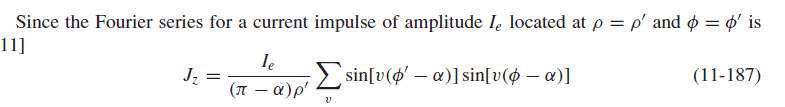# Fourier Series in cylindrical coordinate

## Homework Statement

Here is my question## Homework Equations

I don't know with what formula does the book find fourier series?

## The Attempt at a Solution

Have you tried writing an expression for the current?

Hello deskswirl
Yes , But I know the fourier series for 2-dimensional in cartesian coordinate not cylindrical fourier series.I can do and follow the math procedures.I want to know the main formula that I can derive above equation.

One of the most general forms for the Fourier-Bessel series is given by:
$$\sum\limits_{q}{\sum\limits_{p}{\left\{ \begin{matrix} {{J}_{p}}\left( qr \right) \\ {{Y}_{p}}\left( qr \right)\\\end{matrix} \right\}\left\{ \begin{matrix} \sin \left( p\phi \right) \\ \cos (p\phi ) \\\end{matrix} \right\}\left\{ \begin{matrix} {{e}^{qz}} \\ {{e}^{-qz}} \\\end{matrix} \right\}}}$$
Another is found by letting $$q\to iq$$ in the above expression. These are the two primary solutions of Laplace's equation in circular-cylinder coordinates. Typically due to the symmetry of the problem in phi or z the expression becomes simpler.

•baby_1